## Monday, March 7, 2011

### Jayvee's Great Big Book of Integers

Chapter 1: Grade 7 Integer Review

-A zero pair is a 2 number that has the same value but has opposite signs. Ex. -5 and +5 = 0
-Integers can also be represented on a number line.
-Another way of representing integers are by using colored chips. Red colored chips represents positive numbers and blue colored chips represents negative numbers.

This are the questions from Adding and Subtracting Integers.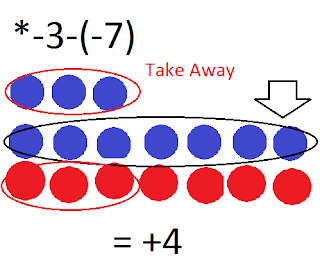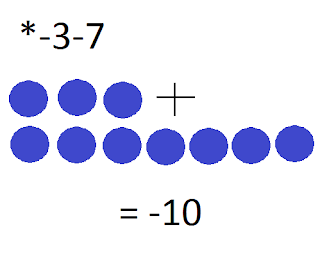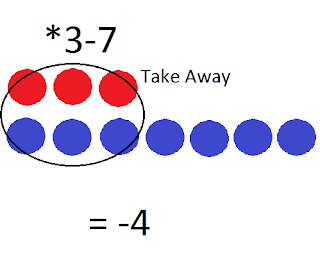Chapter 2: Multiplying Integers

There is only two things to remember when multiplying integers. When you have an even number of negative factors, the product is positive. When you have an odd number of negative factors, the product is negative.

Here are some examples: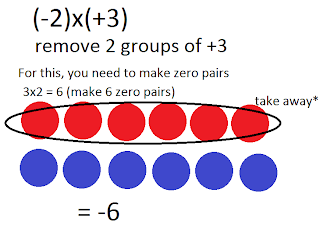Chapter 3: Dividing Integers

Partitive Division is finding how many groups of a number there are between 0 and a number in a number line.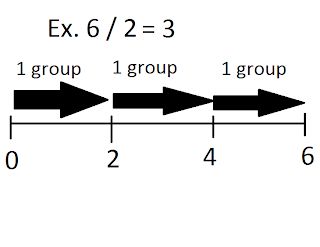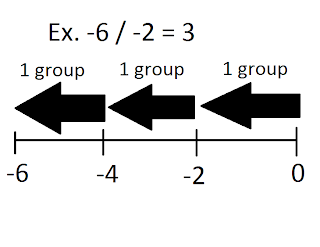Quotative Division is when finding how many objects are in each group then finding how many groups there are.Multiplicative Inverse is when you are finding the quotient of the dividend and the divisor using 2 or 3 ways.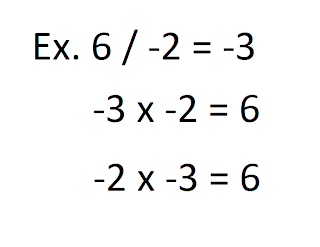Sign Rules :
If there is no negative signs in a question, the answer will be POSITIVE. Ex. 6 / 2 = 3
If there is 1 negative sign in a question the answer will be NEGATIVE. Ex. -6 / 2 = -3
If there is 2 negative signs in a question, the answer will be POSITIVE. -6 / -2 = 3

Chapter 4 : Order of Operations with Integers